# Fraction calculator

The calculator performs basic and advanced operations with fractions, expressions with fractions combined with integers, decimals, and mixed numbers. It also shows detailed step-by-step information about the fraction calculation procedure. Solve problems with two, three, or more fractions and numbers in one expression.

## Result:

### 7/10 - 1/5 = 1/2 = 0.5

Spelled result in words is one half.

### How do you solve fractions step by step?

1. Subtract: 7/10 - 1/5 = 7/10 - 1 · 2/5 · 2 = 7/10 - 2/10 = 7 - 2/10 = 5/10 = 5 · 1/5 · 2 = 1/2
For adding, subtracting, and comparing fractions, it is suitable to adjust both fractions to a common (equal, identical) denominator. The common denominator you can calculate as the least common multiple of both denominators - LCM(10, 5) = 10. In practice, it is enough to find the common denominator (not necessarily the lowest) by multiplying the denominators: 10 × 5 = 50. In the following intermediate step, cancel by a common factor of 5 gives 1/2.
In other words - seven tenths minus one fifth = one half.

#### Rules for expressions with fractions:

Fractions - simply use a forward slash between the numerator and denominator, i.e., for five-hundredths, enter 5/100. If you are using mixed numbers, be sure to leave a single space between the whole and fraction part.
The slash separates the numerator (number above a fraction line) and denominator (number below).

Mixed numerals (mixed fractions or mixed numbers) write as integer separated by one space and fraction i.e., 1 2/3 (having the same sign). An example of a negative mixed fraction: -5 1/2.
Because slash is both signs for fraction line and division, we recommended use colon (:) as the operator of division fractions i.e., 1/2 : 3.

Decimals (decimal numbers) enter with a decimal point . and they are automatically converted to fractions - i.e. 1.45.

The colon : and slash / is the symbol of division. Can be used to divide mixed numbers 1 2/3 : 4 3/8 or can be used for write complex fractions i.e. 1/2 : 1/3.
An asterisk * or × is the symbol for multiplication.
Plus + is addition, minus sign - is subtraction and ()[] is mathematical parentheses.
The exponentiation/power symbol is ^ - for example: (7/8-4/5)^2 = (7/8-4/5)2

#### Examples:

subtracting fractions: 2/3 - 1/2
multiplying fractions: 7/8 * 3/9
dividing Fractions: 1/2 : 3/4
exponentiation of fraction: 3/5^3
fractional exponents: 16 ^ 1/2
adding fractions and mixed numbers: 8/5 + 6 2/7
dividing integer and fraction: 5 ÷ 1/2
complex fractions: 5/8 : 2 2/3
decimal to fraction: 0.625
Fraction to Decimal: 1/4
Fraction to Percent: 1/8 %
comparing fractions: 1/4 2/3
multiplying a fraction by a whole number: 6 * 3/4
square root of a fraction: sqrt(1/16)
reducing or simplifying the fraction (simplification) - dividing the numerator and denominator of a fraction by the same non-zero number - equivalent fraction: 4/22
expression with brackets: 1/3 * (1/2 - 3 3/8)
compound fraction: 3/4 of 5/7
fractions multiple: 2/3 of 3/5
divide to find the quotient: 3/5 ÷ 2/3

The calculator follows well-known rules for order of operations. The most common mnemonics for remembering this order of operations are:
PEMDAS - Parentheses, Exponents, Multiplication, Division, Addition, Subtraction.
BEDMAS - Brackets, Exponents, Division, Multiplication, Addition, Subtraction
BODMAS - Brackets, Of or Order, Division, Multiplication, Addition, Subtraction.
GEMDAS - Grouping Symbols - brackets (){}, Exponents, Multiplication, Division, Addition, Subtraction.
Be careful, always do multiplication and division before addition and subtraction. Some operators (+ and -) and (* and /) has the same priority and then must evaluate from left to right.

## Fractions in word problems:

• In the cafeteriaThere are 18 students in Jacob’s homeroom. Six students bring their lunch to school. The rest eat lunch in the cafeteria. In simplest form, what fraction of students eat lunch in the cafeteria?Add this two mixed numbers: 1 5/6 + 2 2/11=
• A newspaper'sA newspaper's cover page is 3/8 text, and photographs fill the rest. If 2/5 of the text is an article about endangered species, what fraction of the whole cover page is the article about endangered species?
• King's birthday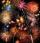To celebrate the king's birthday workers fire 1/5 all purchased rockets. To celebrate the Queen's birthday fire 1/6 of the remaining rockets and to celebrate the birthday of king's son remaining 15,000 rockets. How many rockets they purchased?
• One quarter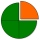Which of the following has a sum of 3/4? A. 1/2+1/4 B. 1/2+1/3 C. 1/4+1/8 D. 1/9+1/12​
• An electricianAn electrician needs 1 1/3 rolls of electrical wire to wire each room in a house. How many rooms can he wire with 6 2/3 rolls of wire?
• A laundryMr. Green washed 1/4 of his laundry. His son washed 3/7 of it. Who washed most of the laundry? How much of the laundry still needs to be washed?
• The tap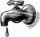For one day flows 148 l of water out of the tap. How much water will flow out for 3/4 day?
• Bitoo and ReenaBitoo ate 3/5 part of an apple and the remaining part was eaten by his sister Reena. How much part of an apple did Renna eat? Who had the larger share? By how much?
• Calories 2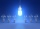Ben eats approximately 2400 calories per day. His wife Sarah eats 5/8 as much. How many calories does Sarah eat per day?
• Orchard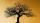3/5 of the trees in the orchard are apples, 1/3 are cherries and the remaining five trees are pear. How many trees are in the orchard?
• Earning 2Rahul earns 5000 Rs, he saves 500 Rs . What's percentage of his earnings does he save? *
• Mountain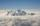Mountain has an elevation of 7450 meters and in the morning is the middle portion thereof in the clouds. How many meters of height is in the sky if below the clouds are 2,000 meters, and above clouds are two-fifths of the mountain's elevation?